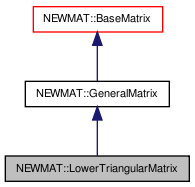# NEWMAT::LowerTriangularMatrix Class Reference

`#include <newmat.h>`

Inheritance diagram for NEWMAT::LowerTriangularMatrix:[legend]

## Detailed Description

Definition at line 752 of file newmat.h.

List of all members.

## Public Member Functions

LowerTriangularMatrix ()
~LowerTriangularMatrix ()
LowerTriangularMatrix (ArrayLengthSpecifier)
LowerTriangularMatrix (const LowerTriangularMatrix &gm)
LowerTriangularMatrix (const BaseMatrix &M)
void operator= (const BaseMatrix &)
LowerTriangularMatrixoperator= (Real f)
LowerTriangularMatrixoperator= (const LowerTriangularMatrix &m)
Real & operator() (int, int)
Real & element (int, int)
Real operator() (int, int) const
Real element (int, int) const
MatrixType Type () const
GeneralMatrixMakeSolver ()
void Solver (MatrixColX &, const MatrixColX &)
LogAndSign LogDeterminant () const
Real Trace () const
void GetRow (MatrixRowCol &)
void GetCol (MatrixRowCol &)
void GetCol (MatrixColX &)
void RestoreCol (MatrixRowCol &)
void RestoreCol (MatrixColX &c)
void NextRow (MatrixRowCol &)
void ReSize (int)
void ReSize (const GeneralMatrix &A)
MatrixBandWidth BandWidth () const
void operator+= (const LowerTriangularMatrix &M)
void operator-= (const LowerTriangularMatrix &M)
void operator+= (Real f)
void operator-= (Real f)
void swap (LowerTriangularMatrix &gm)

## Private Member Functions

GeneralMatrixImage () const

## Constructor & Destructor Documentation

 NEWMAT::LowerTriangularMatrix::LowerTriangularMatrix ( )

Definition at line 756 of file newmat.h.

Referenced by Image().

 NEWMAT::LowerTriangularMatrix::~LowerTriangularMatrix ( )

Definition at line 757 of file newmat.h.

 NEWMAT::LowerTriangularMatrix::LowerTriangularMatrix ( ArrayLengthSpecifier n )

Definition at line 63 of file newmat4.cpp.

 NEWMAT::LowerTriangularMatrix::LowerTriangularMatrix ( const LowerTriangularMatrix & gm )

Definition at line 759 of file newmat.h.

 NEWMAT::LowerTriangularMatrix::LowerTriangularMatrix ( const BaseMatrix & M )

Definition at line 137 of file newmat4.cpp.

## Member Function Documentation

 MatrixBandWidth NEWMAT::LowerTriangularMatrix::BandWidth ( ) const` [virtual]`

Reimplemented from NEWMAT::BaseMatrix.

Definition at line 451 of file newmat4.cpp.

 Real NEWMAT::LowerTriangularMatrix::element ( int m, int n ) const

Definition at line 717 of file newmat6.cpp.

 Real & NEWMAT::LowerTriangularMatrix::element ( int m, int n )

Definition at line 709 of file newmat6.cpp.

 void NEWMAT::LowerTriangularMatrix::GetCol ( MatrixColX & mrc ) ` [virtual]`

Implements NEWMAT::GeneralMatrix.

Definition at line 367 of file newmat3.cpp.

 void NEWMAT::LowerTriangularMatrix::GetCol ( MatrixRowCol & mrc ) ` [virtual]`

Implements NEWMAT::GeneralMatrix.

Definition at line 342 of file newmat3.cpp.

 void NEWMAT::LowerTriangularMatrix::GetRow ( MatrixRowCol & mrc ) ` [virtual]`

Implements NEWMAT::GeneralMatrix.

Definition at line 335 of file newmat3.cpp.

 GeneralMatrix * NEWMAT::LowerTriangularMatrix::Image ( ) const` [private, virtual]`

Reimplemented from NEWMAT::GeneralMatrix.

Definition at line 781 of file newmat4.cpp.

 LogAndSign NEWMAT::LowerTriangularMatrix::LogDeterminant ( ) const` [virtual]`

Reimplemented from NEWMAT::GeneralMatrix.

Definition at line 659 of file newmat8.cpp.

 GeneralMatrix* NEWMAT::LowerTriangularMatrix::MakeSolver ( ) ` [virtual]`

Reimplemented from NEWMAT::GeneralMatrix.

Definition at line 773 of file newmat.h.

 void NEWMAT::LowerTriangularMatrix::NextRow ( MatrixRowCol & mrc ) ` [virtual]`

Reimplemented from NEWMAT::GeneralMatrix.

Definition at line 393 of file newmat3.cpp.

 Real NEWMAT::LowerTriangularMatrix::operator() ( int m, int n ) const

Definition at line 167 of file newmat6.cpp.

 Real & NEWMAT::LowerTriangularMatrix::operator() ( int m, int n )

Definition at line 55 of file newmat6.cpp.

 void NEWMAT::LowerTriangularMatrix::operator+= ( Real f )

Reimplemented from NEWMAT::GeneralMatrix.

Definition at line 788 of file newmat.h.

 void NEWMAT::LowerTriangularMatrix::operator+= ( const LowerTriangularMatrix & M )

Definition at line 786 of file newmat.h.

Referenced by operator+=().

 void NEWMAT::LowerTriangularMatrix::operator-= ( Real f )

Reimplemented from NEWMAT::GeneralMatrix.

Definition at line 789 of file newmat.h.

 void NEWMAT::LowerTriangularMatrix::operator-= ( const LowerTriangularMatrix & M )

Definition at line 787 of file newmat.h.

Referenced by operator-=().

 LowerTriangularMatrix& NEWMAT::LowerTriangularMatrix::operator= ( const LowerTriangularMatrix & m )

Definition at line 763 of file newmat.h.

 LowerTriangularMatrix& NEWMAT::LowerTriangularMatrix::operator= ( Real f )

Reimplemented from NEWMAT::GeneralMatrix.

Definition at line 762 of file newmat.h.

 void NEWMAT::LowerTriangularMatrix::operator= ( const BaseMatrix & X )

Definition at line 401 of file newmat6.cpp.

Referenced by operator=().

 void NEWMAT::LowerTriangularMatrix::ReSize ( const GeneralMatrix & A ) ` [virtual]`

Reimplemented from NEWMAT::GeneralMatrix.

Definition at line 356 of file newmat4.cpp.

 void NEWMAT::LowerTriangularMatrix::ReSize ( int nr )

Definition at line 266 of file newmat4.cpp.

Referenced by ReSize().

 void NEWMAT::LowerTriangularMatrix::RestoreCol ( MatrixColX & c ) ` [virtual]`

Reimplemented from NEWMAT::GeneralMatrix.

Definition at line 781 of file newmat.h.

Referenced by RestoreCol().

 void NEWMAT::LowerTriangularMatrix::RestoreCol ( MatrixRowCol & mrc ) ` [virtual]`

Reimplemented from NEWMAT::GeneralMatrix.

Definition at line 383 of file newmat3.cpp.

 void NEWMAT::LowerTriangularMatrix::Solver ( MatrixColX & mcout, const MatrixColX & mcin ) ` [virtual]`

Reimplemented from NEWMAT::GeneralMatrix.

Definition at line 71 of file newmat7.cpp.

 void NEWMAT::LowerTriangularMatrix::swap ( LowerTriangularMatrix & gm )

Definition at line 790 of file newmat.h.

Referenced by NEWMAT::swap().

 Real NEWMAT::LowerTriangularMatrix::Trace ( ) const` [virtual]`

Reimplemented from NEWMAT::BaseMatrix.

Definition at line 567 of file newmat8.cpp.

 MatrixType NEWMAT::LowerTriangularMatrix::Type ( ) const` [virtual]`

Implements NEWMAT::GeneralMatrix.

Definition at line 430 of file newmat4.cpp.

The documentation for this class was generated from the following files:

 newmat11b Generated Mon May 9 04:54:19 2016 by Doxygen 1.6.3